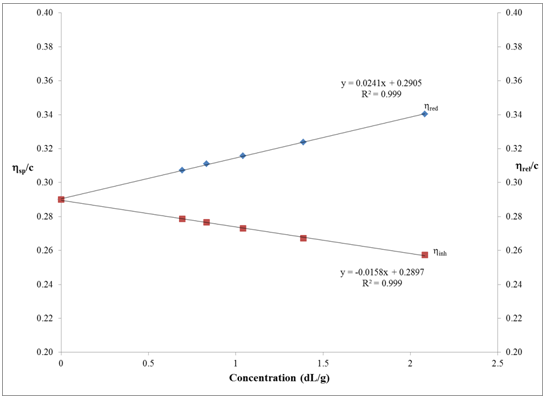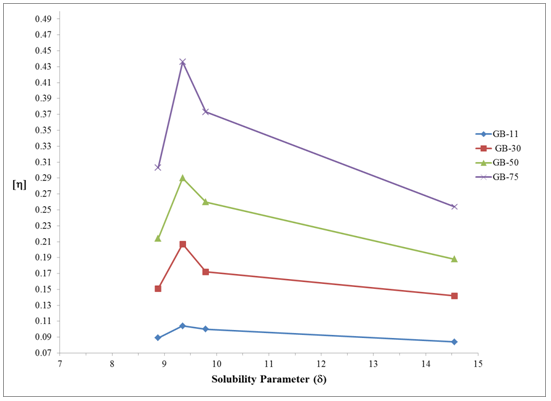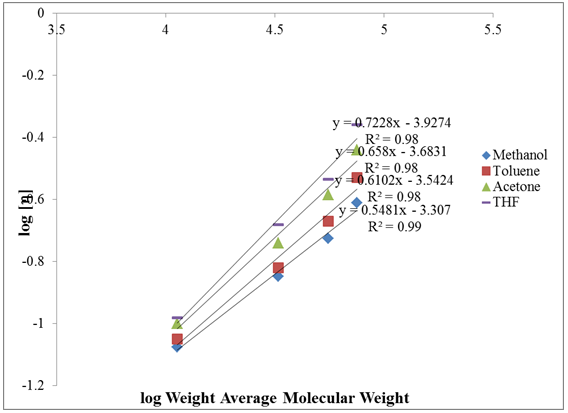## Figures index

#### Ronald P. D’Amelia, Jaksha C. Tomic, William F. Nirode

Journal of Polymer and Biopolymer Physics Chemistry. 2014, 2(4), 67-72 doi:10.12691/jpbpc-2-4-2• Figure 1. Intrinsic Viscosity Plot of ηred and ηinh vs Concentration for PVAc GB-50 in THF is shown. Extrapolation of the Huggins (ηred) and the Kraemer (ηinh) lines gives the intrinsic viscosity for the polymer• Figure 2. Plot of [η] vs solubility parameter (δ) for GB-11, GB-30, GB-50, and GB-75 are shown. The peak in each plot corresponds to the maximum intrinsic viscosity in THF• Figure 3. A double log plot of [η] vs Mw is shown. The slope is equal to the α and the intercept is equal to K, constants of the Mark Houwink equation